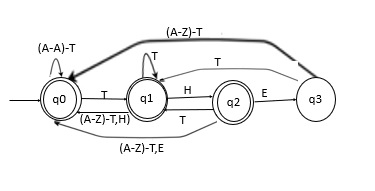# Construct DFA for strings not ending with "THE"

A Deterministic Finite automaton (DFA) is a five tuples

M=(Q, Σ, δ,q0,F)

Where,

• Q : Finite set called states.
• Σ : Finite set called alphabets.
• δ : Q × Σ → Q is the transition function.
• q0 ϵ Q is the start or initial state.
• F : Final or accept state.

## Accept Strings which are not ending with "THE"

• Observe whether the given string is ending with “the” or not.

• The different notations of “the” which are avoided in the end of the string are as follows −

"tHE", "thE", "THE", "ThE", "THe", "The", "tHe" and "the"

• These all strings are not accepted from alphabet (A-Z)

Let the initial state is q0Consider 4 states q0, q1, q2 and q3.

Let’s take a variable named DFA which will be initially 0.

Whenever a transition is there, it will update the value of DFA with the number associated with the new state.

## Example

• If a transition occurs from state q0 to state q1 then the value of DFA will be updated to 1.

• If a transition occurs from state q2 to state q3 then the value of DFA will be updated to 3.

• In this way, apply this algorithm on the entire string and if it reaches the end, then reach state 0, 1 or 2. Thus, our string will be accepted. Otherwise, it won’t be accepted.

Case 1

Input − pQdfGTthe

Output − Not Accepted

Case 2

Input − ThesdGTYid

Output − ACCEPTED